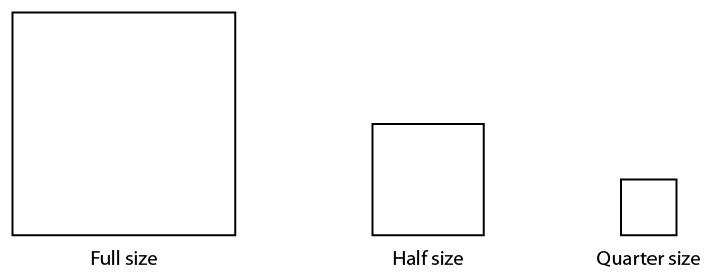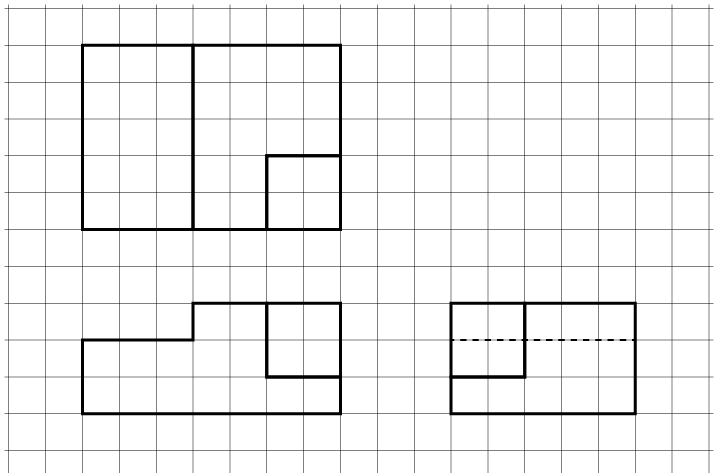# 6.1: Sketching Technique

Sketching technique

Sketching provides a quick and simple way to express ideas and to communicate the shape and general size of an object.

Sketching parallel lines

Start by drawing lines that are parallel to the edges of the paper, such as a border line and title block. Use your finger as a guide when you draw along the grid line on the sketch pad (Figure 1). If you let the end of your little finger run down the edge of the paper pad as you draw, this will steady your hand and make it easier to get a straight line.1. Sketching a parallel vertical line

Sketching non-parallel lines

When you are sketching lines that are not parallel to the sides of the paper, turn the paper around so that the line you wish to draw is either straight up and down in front of you or straight across the sheet of paper.

It is much easier to draw lines this way, rather than at an angle across the sheet. Let the side of your little finger rest on the paper as you draw. This will help you steady your hand (Figure 2).2. Sketching a horizontal line

Sketching a rectangle

Locate the corners of the rectangle first. Then place your paper in a comfortable position for sketching and sketch downward for vertical lines and left to right for horizontal lines. Use the grid lines as a guide to maintain lines parallel and at 90 degrees to each other (Figure 3).3. Sketching a rectangle

Sketching a circle

First, locate the centre of the circle (Figure 4), and then very lightly box in the size of the circle (using the diameter as a guide), as in the top right. Sketch in the circle, one quarter at a time, as shown in the bottom row, left to right. You may find it necessary at first to add light points along the projected circumference to help guide you through each quarter. Remember to move your sketch pad so that you can maintain a comfortable sketching position.4. Sketching a circle

Sketching to approximate scale

In Figure 5, the full-size square is on the left. The centre square is half size, and the right square is quarter size. Note that centre and right squares are the same shape as the left square, only smaller.5. Sketching to scale

When you are sketching freehand, your sketches should reflect the true shapes of objects as much as possible. If you use grid paper, it is not difficult to sketch to an approximate scale. Assume that the object in Figure 6 is shown full size. As it is necessary to show all orthographic views on the same sheet of paper, the views must be scaled. Figure 7 shows the views at approximately one-half the original size.6. Full-size isometric object7. Scale orthographic projections